Programming Fundamentals
Lecture 01 Introduction to Programming
Edirlei Soares de LimaComputer Programs
Programs are created to solve problems and perform specific
Example: collect the items to complete the level
Player
Items
Bag
Bag slots:
Problem: how to know when the player has completed the level?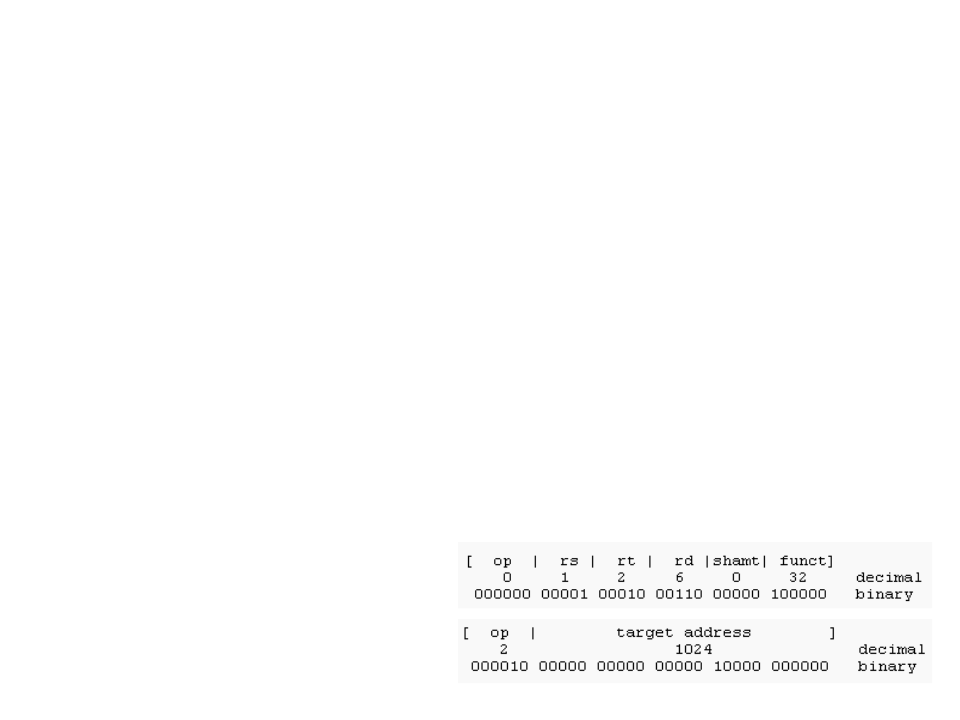Machine Code
A computer's central processing unit (CPU) only runs machine
code.
Machine code is a strictly numerical language and is intended to run as
fast as possible.
The instructions causes the CPU to perform specific task, such
arithmetic operations and registry manipulations.
Machine code is represented by sequences of binary digits.
Exemple:
Adding the registers 1 and 2
and placing the result in
register 6.High-Level Programming Languages
While it is possible to write programs directly in machine
code, it is very complex to manage individual bits and
Today, the vast majority of programs are written in high-level
programming languages.
In a high-level language, instead of dealing with registers and
functions, loops, boolean expressions, arrays, etc.Popular Programming LanguagesExample of Code in Lua
local cels
local fahr
io.write("Temperature in Celsius: ")
fahr = 1.8 * cels + 32
io.write("Temperature in Fahrenheit: ", fahr, "\n")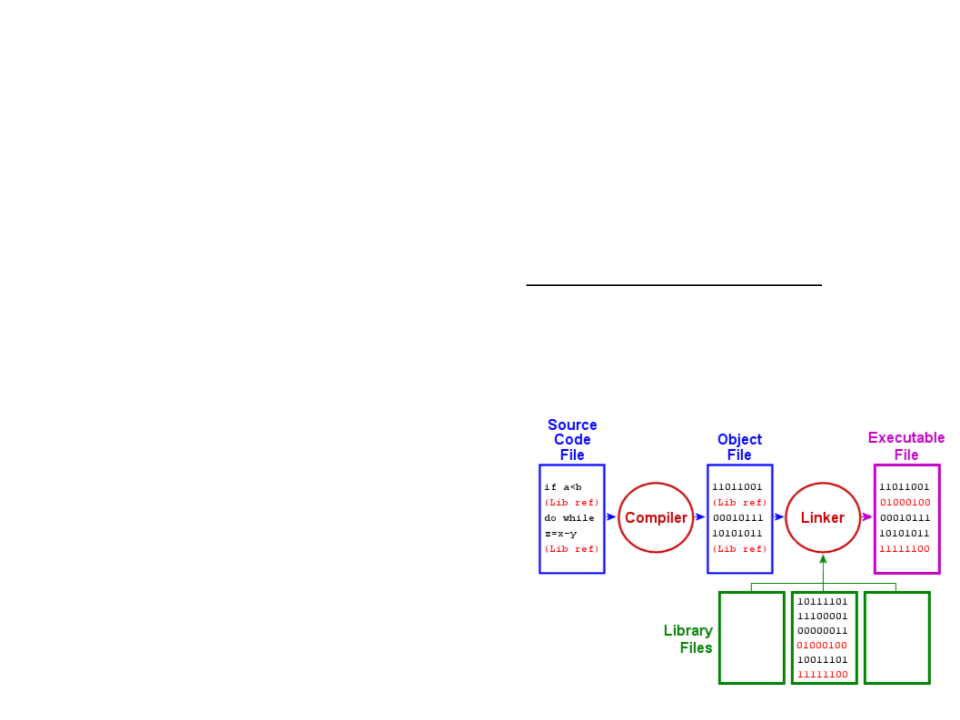Compilation Process
Programs written in high-level languages cannot be directly
executed in the CPU.
Before running the code, it must be translated to machine code.
This task (compilation) is performed by a program called
compiler.
Examples of compiled languages:
C, C++, Basic, Pascal, …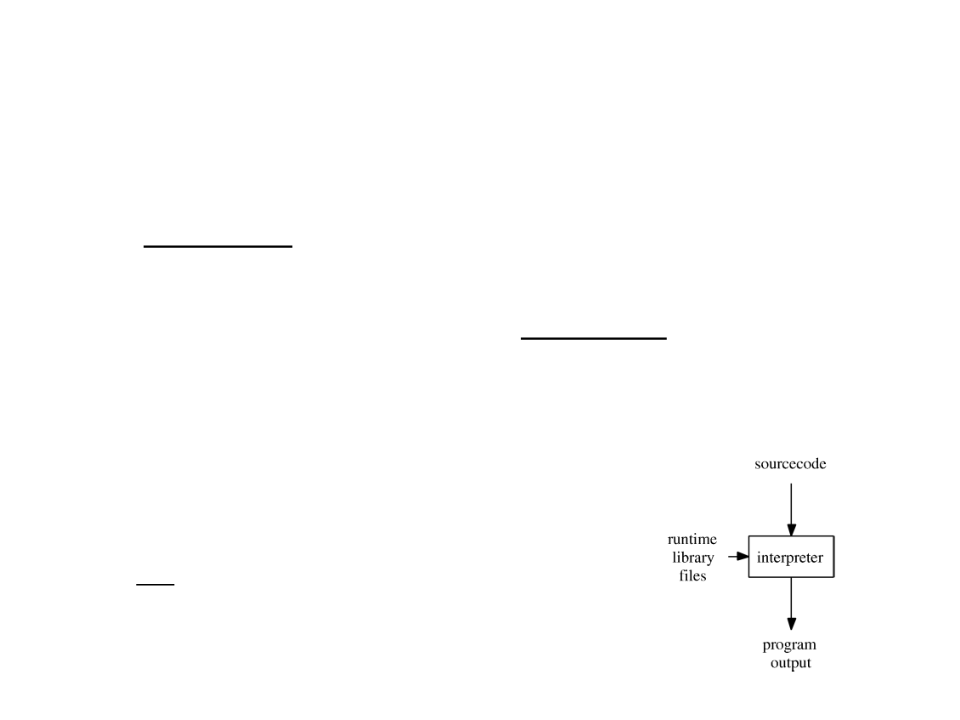Interpreted Programming Languages
Not all programming languages are compiled. Some of them
are interpreted.
An interpreted language uses an interpreter program to
execute the code directly, translating each statement into a
sequence of one or more operations that are performed by
the interpreter.
Examples of interpreted languages:
Lua, Java, JavaScript, Python, PHP, …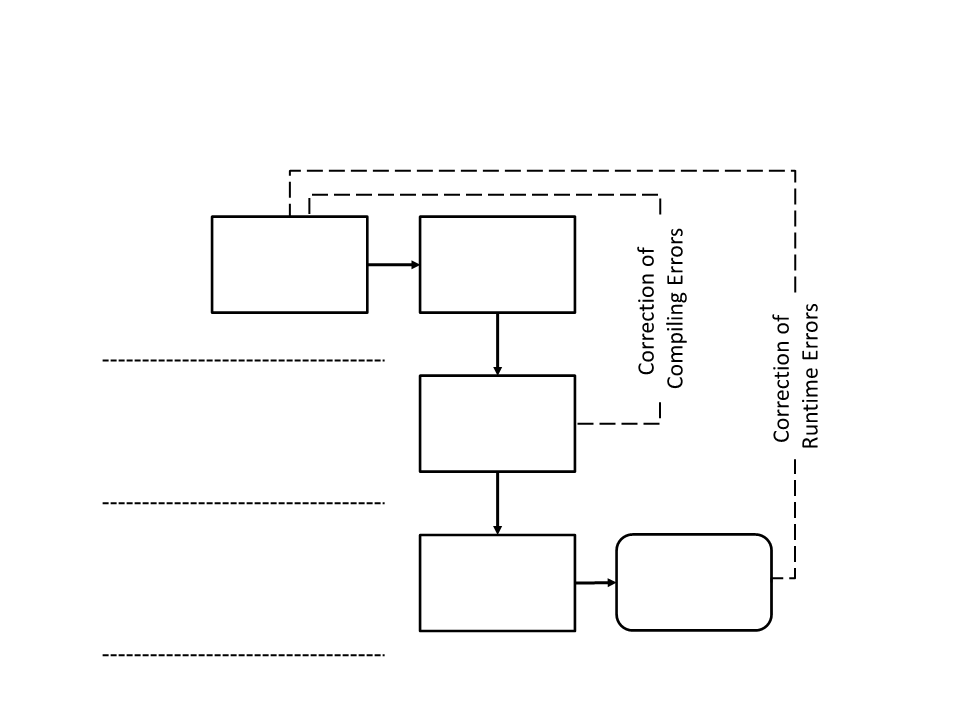Development Cycle (Compiled)
Program
Text Editor
Code
Development
Compiler
Compilation
Program
Output
Test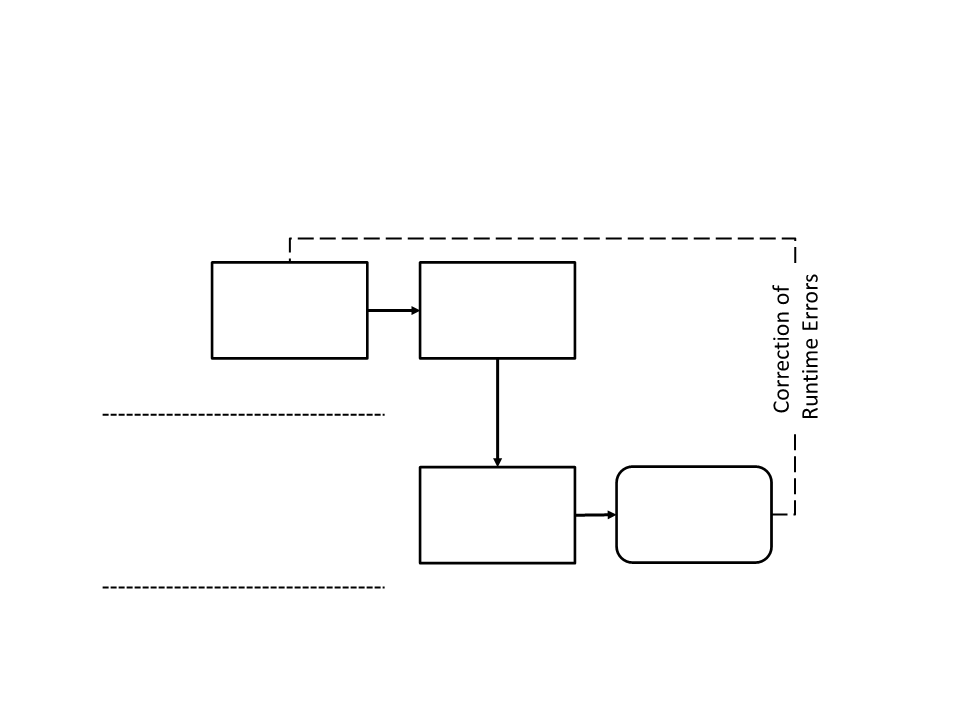Development Cycle (Interpreted)
Program
Text Editor
Code
Development
Interpreter
Output
Test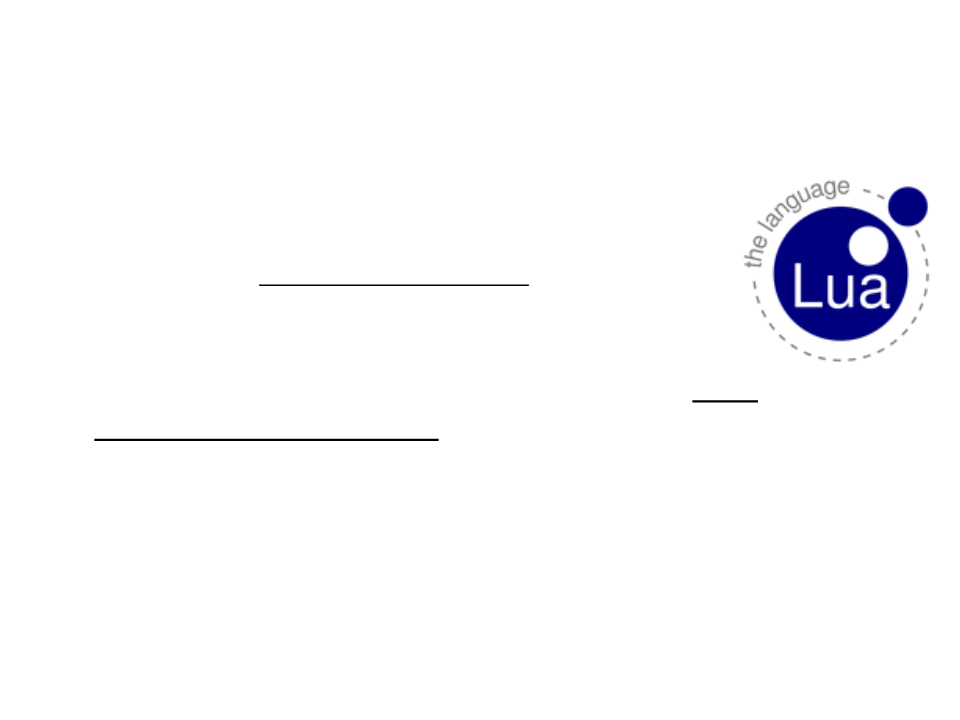Lua Programming Language
Lua is a powerful, efficient, lightweight,
embeddable scripting language.
It supports procedural programming, object-oriented
programming, functional programming, ...
In video game development, Lua is one of the most
popular scripting language for game programming.
Some games that use Lua: World of Warcraft, Civilization
V, Far Cry, Angry Birds, Grim Fandango, Dota 2, ...
Lua is designed, implemented, and maintained by
a team of researchers at PUC-Rio in Brazil.Why choose Lua?
Lua is a proven, robust language:
Has been used in many industrial applications (e.g.: Adobe's Photoshop
Lightroom) and games (e.g.: World of Warcraft and Angry Birds).
Is the leading scripting language in games and won the Front Line
Award 2011 from the Game Developers Magazine.
Has a solid reference manual and several books.
Lua is fast:
Lua has a deserved reputation for performance. Several benchmarks
show Lua as the fastest language in the realm of interpreted scripting
languages.Why choose Lua?
Lua is portable:
is distributed in a small package and builds out-of-the-box in all
platforms that have a standard C compiler.
Lua runs on Unix, Windows, mobile devices (Android, iOS, BREW,
Symbian, Windows Phone), on embedded microprocessors, etc.
Lua is embeddable:
Lua can be easily embedded into other applications. Lua API allows
strong and easy integration with code written in other languages.
Lua is free:
Lua is a free open-source software that can be used for any purpose,
including commercial purposes, at absolutely no cost.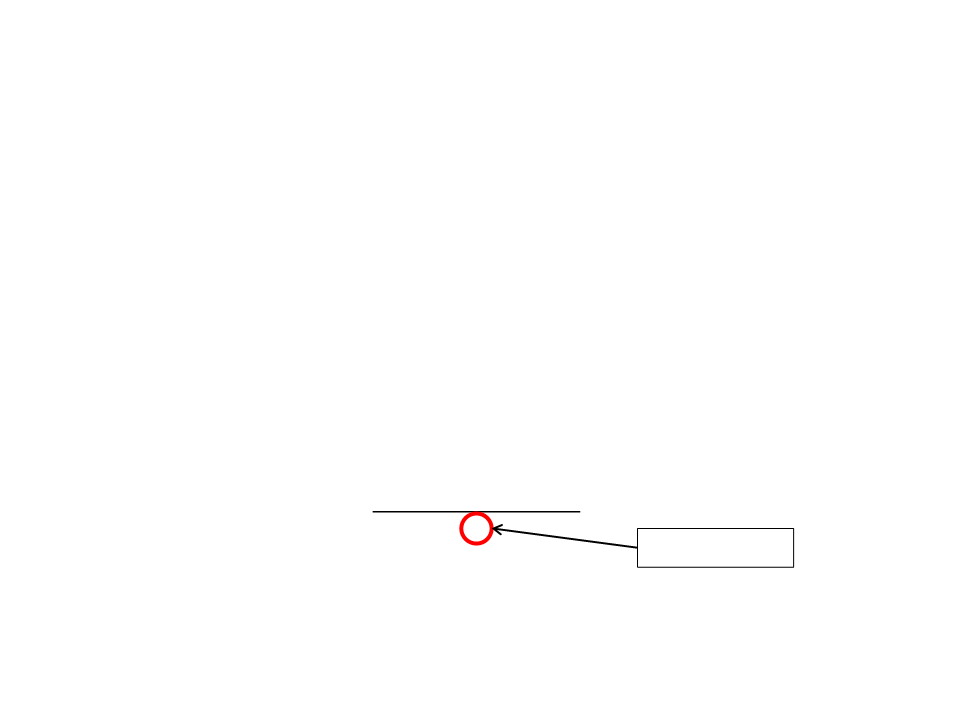Variables and Constants
Variables and constants are the basic elements
manipulated by a program.
Constant is a fixed value that doesn’t change during
the execution of a program.
ꢅꢆꢃꢇꢈ1 + ꢅꢆꢃꢇꢈ2
ꢁꢂꢃꢄꢅꢆꢃꢇꢈ =
2
Contant value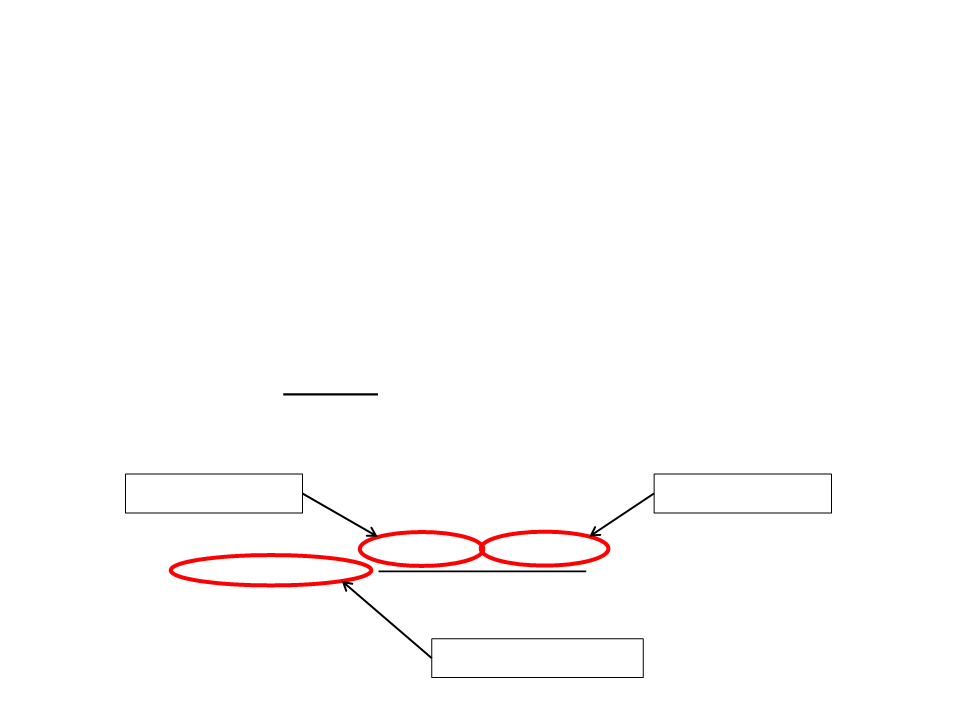Variables
Variable is a space in the memory of the computer that is
reserved to store a specific type of data.
Containers where we can store information (numbers, text, etc.)
Variables have names so they can be referenced in the code
and have their values accessed or changed when necessary.
ꢅꢆꢃꢇꢈ1 + ꢅꢆꢃꢇꢈ2
ꢁꢂꢃꢄꢅꢆꢃꢇꢈ =
2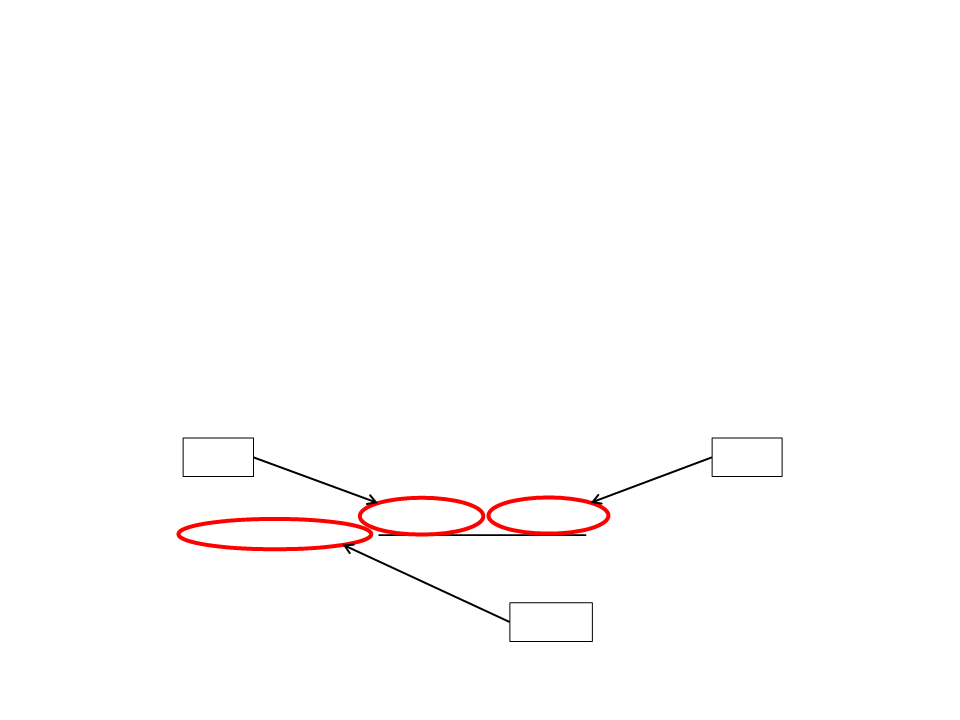Variables
The content of a variable can change during the execution of a
program.
Although different values can be assigned to the same variable,
it can only store one value at a time.
“7.5”
“8.0”
ꢅꢆꢃꢇꢈ1 + ꢅꢆꢃꢇꢈ2
ꢁꢂꢃꢄꢅꢆꢃꢇꢈ =
2
“7.75”Variables
Variables have:
Name: used to refer to the variable in the code;
Name restrictions: is not allowed to start the name of variable with a number (0-9),
some special characters are not allowed in the names (*, -, /, +, ...), and some
reserved word can not be used as well (if, for, while, ...).
Type: defines the set of values that can be stored in the variable;
Value: the value stored;
Variables must be:
Declared: What is the name and what is the type of the variable?
Initialized: What is the initial value of the variable?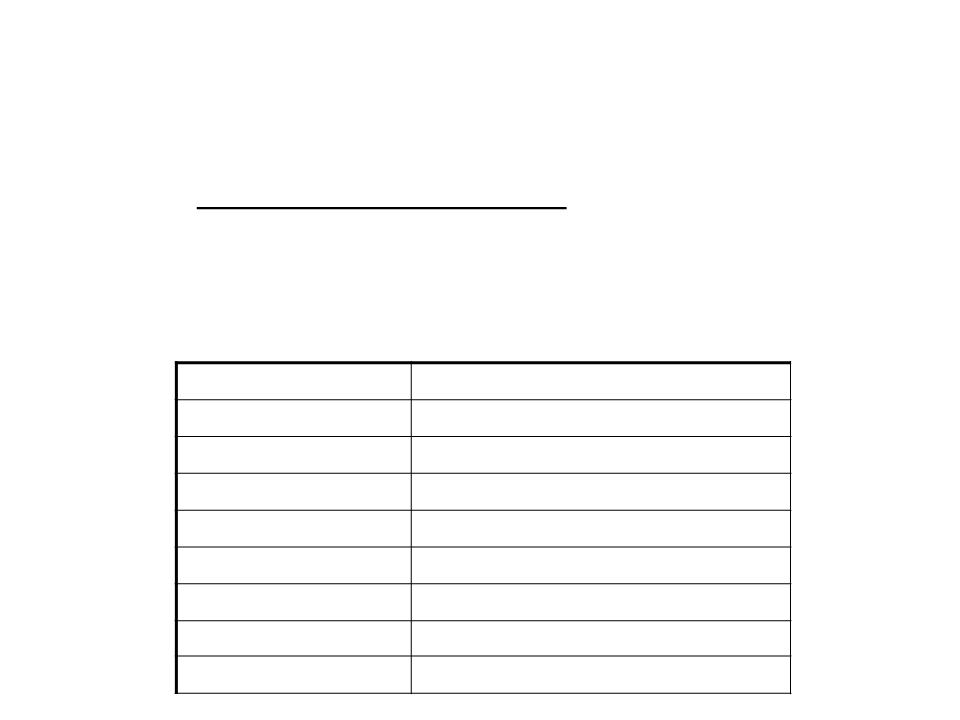Variables in Lua
Lua is a dynamically typed language. This means that when a
variable is declared, its type doesn’t need to be specified.
There are no type definitions in Lua;
Each value carries its own type.
Type
Examples of Values
number
string
0, 1, 2.3, -2.3
hi”, “hello world”,test 123”, “”
true, false
boolean
function
table
0x1234567
0x2345678
userdata
nil
0x3456789
0x4567890
nilDeclaring Variables in Lua
Local variables must be explicitly declared;
More than one variable can be declared at a time;
Variables can be used without being declared (global variables);
Examples:
local a -- declares a local variable called a
local b -- declares a local variable called b
local d, e -- declares two local variables (a and b)
local d = 5 -- declares and initializes the variable d
f = 10 -- initializes a global variable f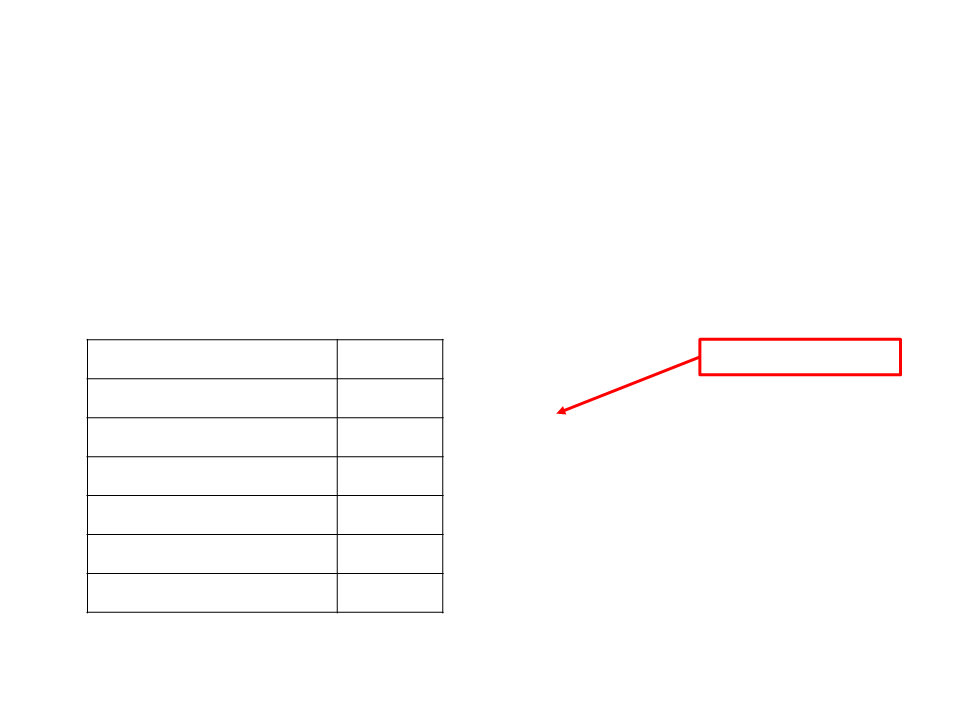Arithmetic Operators
Arithmetic Operators are used to perform arithmetic
operations with variables and constants.
Operation
Symbol
Examples:
assignment operator
+
-
total = price * quantity
result = 3 * (1 - 2) + 4 * 2
res = 4 % 2
Subtraction
Multiplication
Division
*
/
Remainder or modulus
Exponentiation
%
^
res = b ^ 2Input and Output Functions
Function writeof the io” module: is used to write
data to the output console.
io.write(constants/variables/expressions...)
io.write(33)
Output:
33
local myVar = 5
io.write("Value = ", 33, " Total = ", 33 + 40, " Var = ", myVar)
Output:
Valor = 33 Total = 73 Var = 5Input and Output Functions
Text output:
io.write("Programming Fundamentals\nwith Lua")
Output:
Programming Fundamentals
with LuaInput and Output Functions
the console (keyboard input).
local n
The value typed by the user is stored in the variable n
Important: the input value is always a string (text).
Sometimes you need to convert the value to a number type
with the function tonumber:
local n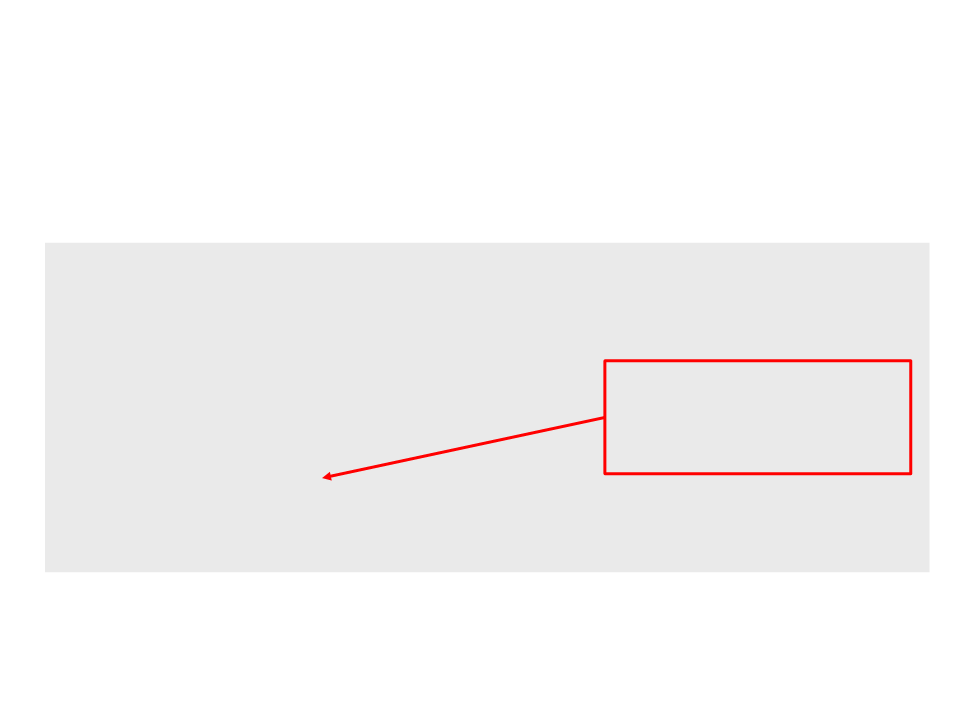Example 1
Problem: read two numbers and show the sum of the numbers.
local number1, number2, result
io.write("First number: ")
In this case, we don’t need to
convert the values to numbers
because arithmetic operators
automatically convert them.
io.write("Second number: ")
result = number1 + number2
io.write("The sum is ", result)Lua Programming - Example
-- Program to convert temperatures from Celsius to Fahrenheit
local cels -- variable to store the temperature in Celsius
local fahr -- variable to store the temperature in Fahrenheit
io.write("Temperature in Celsius: ")
fahr = 1.8 * cels + 32 -- convert from Celsius to Fahrenheit
-- Show the temperature in Fahrenheit
io.write("Temperature in Fahrenheit: ", fahr, "\n")Exercise 1
a) Write a program that converts kilometers per hour to miles
per hour (1 km/h is equal to 0.6213711922 mi/h).
b) Write a program that calculates the perimeter of a rectangle
(ꢉ = 2 ꢄꢈꢂꢊꢋꢌ + ꢍꢁꢇꢌꢋ ).
c) Write a program that takes hours and minutes as input, and
calculates the total number of minutes.
d) Write a program that takes minutes as input, and displays
the total number of hours and minutes.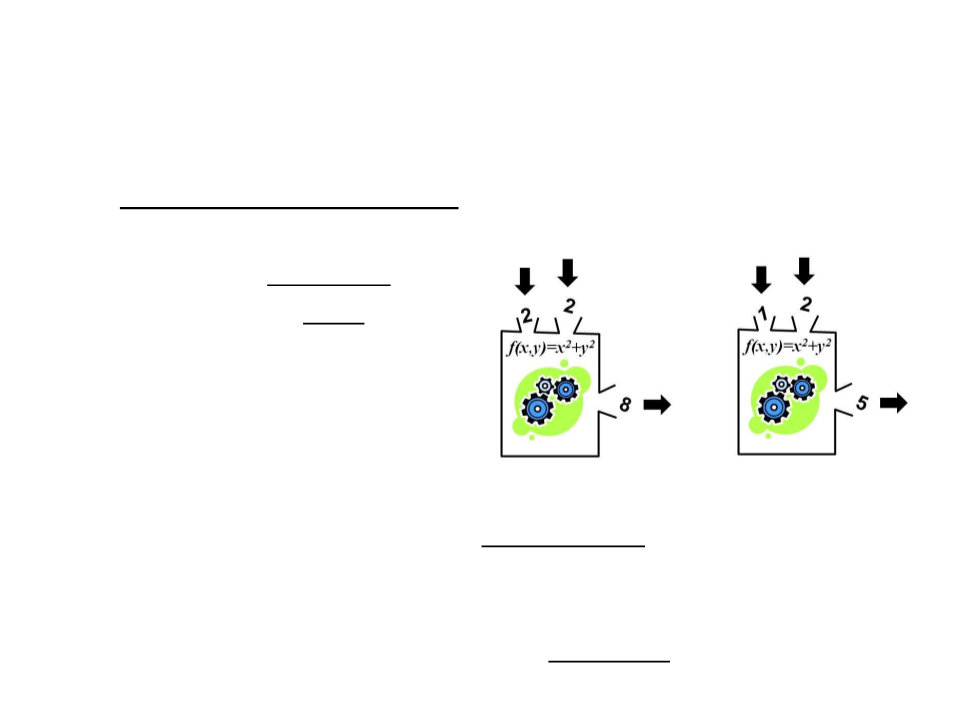Functions
A function is a block of code with a name that can be executed
at other points in the code.
It may have parameters
It may return a result
Functions are important to:
Simplify and organize the code (modularization);
Avoid repetitions of code;
Extend the programming language;
Once declared, we can just use them (abstraction)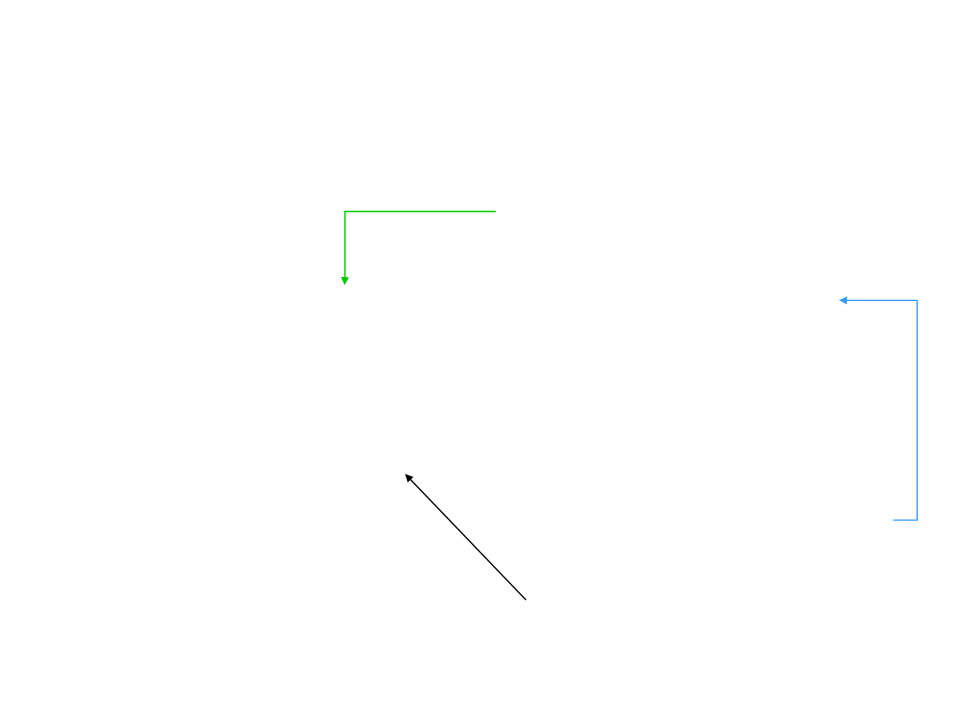Functions in Lua
A Lua program cannot have two
functions with the same name.
function function_name(parameter1, parameter2)
local variables
Lua instructions
return
If a function doesn’t have
end
parameters, we just use: ()
Block of codeFunctions in Lua Example
function celsius_fahrenheit(tc)
local f
f = 1.8 * tc + 32
return f
end
local cels, fahr
io.write("Temperatura in Celsius: ")
fahr = celsius_fahrenheit(cels)
io.write("Temperature in Fahrenheit: ", fahr)
We can use the functioncelsius_fahrenheit in any other
program where this conversion may be needed.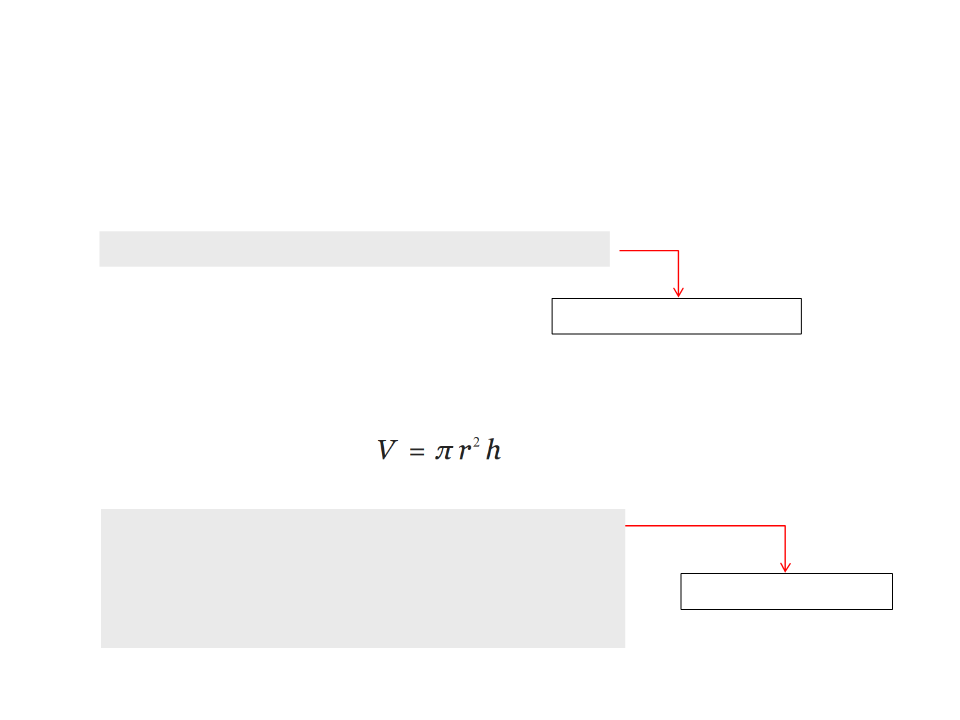Parameters and Return Values
Example:
function celsius_fahrenheit(tc)
Only one input parameter.
Example of function with two parameters:
Volume of a cylinder:
function volume_cylinder(r, h)
local v
v = math.pi * (r ^ 2) * h
return v
end
Two input parametersParameters and Return Values
function volume_cylinder(r, h)
local v
v = math.pi * (r ^ 2) * h
return v
end
io.write("Height of the cylinder: ")
io.write("Volume of the cylinder: ", volume)Scope of Variables
The scope of a variable is the region of the program where the
variable is valid/exist.
A variable declared inside the block of code of a function with
the keyword “local” is a local variable:
The variable is only valid inside the block of code of the function
where it was declared.
When the execution of the function ends, the memory area reserved
to store local variables is automatically released, so the program can
no longer access those variables.Scope of Variables
Local variable:
A function can be executed multiple times.
For each execution, new memory areas for local variables are
automatically reserved. When the function ends, the memory is
automatically released.
Local variables declared inside the block of code of a
function are not valid in other functions.
The parameters of a function are also local variables that
are only valid in the block of code of the function.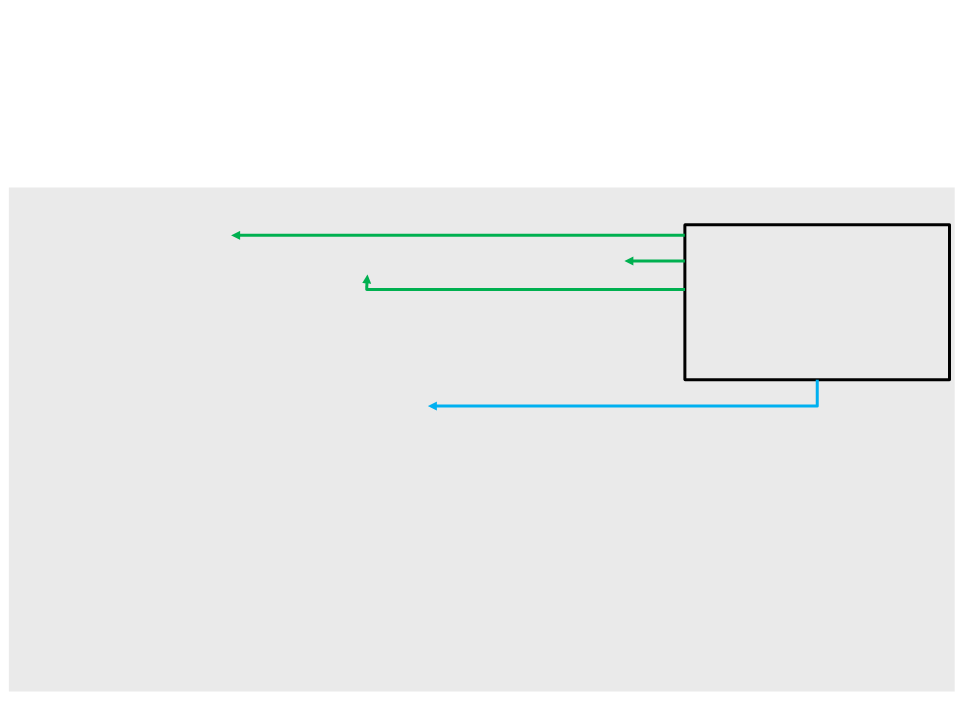Scope of Variables
local volume
volume = math.pi * (radius ^ 2) * height
return volume
end
Variables with the same
name, but with different
scopes.
io.write("Height of the cylinder: ")
io.write("Volume of the cylinder: ", volume)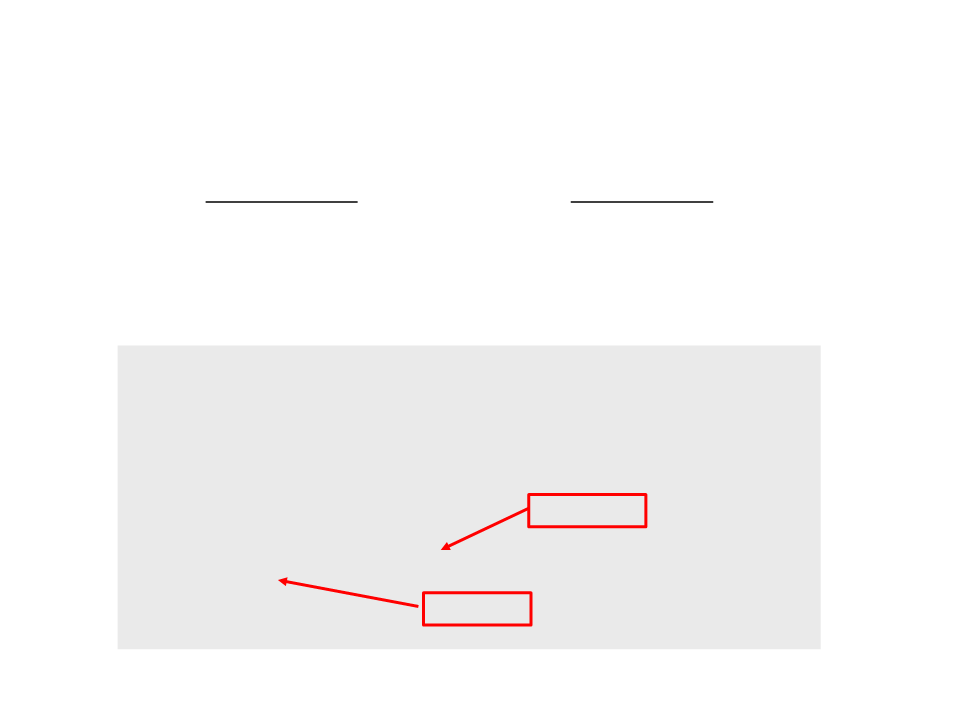Scope of Variables
Functions receive values as parameters and return values (not variables).
The name of the variables may be the same, but they are different
variables.
function doble_value(x)
x = x * 2
return x
end
Output: 10.0
local x = 5.0
io.write(doble_value(x))
io.write(x)
Output: 5.0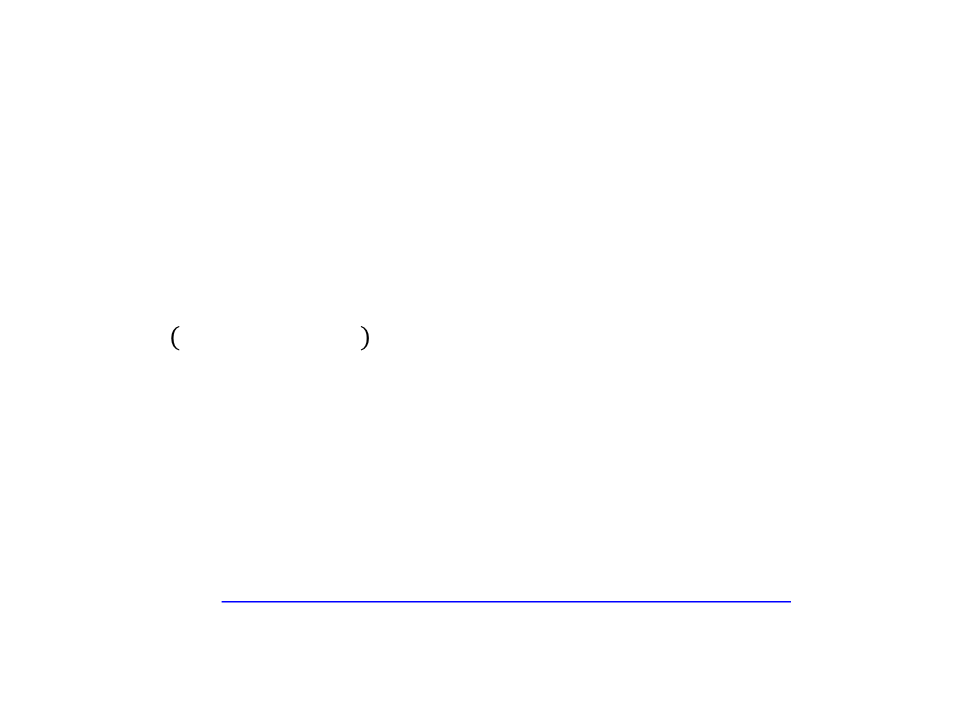Exercise 2
2
) Rewrite the first exercises using functions:
a) Write a program that converts kilometers per hour to miles per hour
(
1 km/h is equal to 0.6213711922 mi/h).
b) Write a program that calculates the perimeter of a rectangle ( =
ꢄꢈꢂꢊꢋꢌ + ꢍꢁꢇꢌꢋ ).
2
c) Write a program that takes hours and minutes as input, and
calculates the total number of minutes.
d) Write a program that takes minutes as input, and display the total
number of hours and minutes.
Extra Exercises: https://edirlei.com/aulas/gameprog/ExtraExercisesLua.pdf (optional)